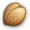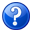# Call of Duty 5: Dynamic FoliageJump to: navigation, searchThis tutorial will show how to create Dynamic Foliage in COD:WAW. Credits for Tutorial and Script goes to Zweimann

# Intro

By Zweimann
This is an easy way to have Dynamic Foliage in your custom map. Only 2 Steps. This code works and needs no mod, so your map runs dynamic foliage as a standalone block.
This is what you get:

# Preparation and Radiant

• Change your misc_models (Trees and Palms, Foliage and grass) to script_models.
• Choose between 3 different targetnames for them (can be any of them but changes must be reflected in the script in next Step):

Trees and palms:

`targetname: dyn_trees`

Big Foliage:

`targetname: dyn_foliage`

Grass and tiny foliage:

`targetname: dyn_grass`

## TIPS

It can be a long task to change all your misc_models into script_model and add the targetname so here is one way to do it quicker:Make a backup of your .MAP files before starting this Tutorial in case something goes wrong!

• Select one of the model you wish to have dynamic - for this example lets say the model is called foliage_cod5_tallgrass10a
• Bring up the Entity properties using n
• Highlight the K/V Model/foliage_cod5_tallgrass10a
• Close the Entity properties by pressing n again
• Now go to Selection > Select by Key/Value (or use shorcut SHIFT-CRTL-F):
• After you click you will see that the Key Model and Value foliage_cod5_tallgrass10a will be already filled in, click OK
• This will now select all the other Model called foliage_cod5_tallgrass10a in the level
• Bring up the Entity properties using n
• Now you can change misc_model Key to script_model (highlight it first then edit)
• After this you can add the new K/V:
```Key: Targetname
Value: Dyn_foliage
```Script_models do not support Modelscale!! Make sure to reset to 1 or remove the modelscale K/V if any and replace the models accordingly on ground.

• You can now press Enter to complete and keep going with other models
• Remember to keep an eye on the number of script_model using M:

# Scripting

• Create a new file in raw/maps/mp/ called _dynamic_foliage.gsc
• Open it with Notepad and copy/paste the following script:
```///////////////////////////////////////////////////////////
// Dynamic Foliage v.1 for CoD:WaW & CoD:MW
// Coded by Zweimann ( [email protected] )
// This code has been written to enhance the ingame experience,
// adding dynamic scenarios instead of static ones.
///////////////////////////////////////////////////////////
#include maps\mp\_utility;
#include common_scripts\utility;

// ---------------------------------------------------------------------
// Init Script
// ---------------------------------------------------------------------

initdfs()
{
println("^1Initializing Zweimann's Dynamic Foliage System");

// There should be three different script_models: trees, foliage and grass, because they're
// treated in a different way.
// Consider Grass to grass (obviously) and to very small foliage elements
// Consider Foliage to midsize foliage
// classname: script_model
// targetname: <name>
// These names were used in Burma 1.1. Change them to whatever you want
level.dfs_tree_sm_name = "dyn_trees";
level.dfs_foliage_sm_name = "dyn_foliage";
level.dfs_grass_sm_name = "dyn_grass";

// You must set up here Strength of Wind, to simulate higher or lower sway
// on foliage and trees.
// 0 means no wind @ all
// 10 means A HURRICANE : )
// Recommended values: 0.3 to 0.6. Optimal value: 0.5;
// Obviously you can setup a dvar or whatever for this

level.dfs_wind_strength = 0.5;
// Let's start

foliage_animation();
grass_animation();
tree_animation();
}

// -----------------------------------------------------------------
// Main Scripts
// -----------------------------------------------------------------

tree_animation()
{
level endon("game_ended");
// Sway animation for Trees
// Slower, softer

entities = getentarray( level.dfs_tree_sm_name, "targetname" );

vpoint = level.dfs_wind_strength / 1.4;
vangles = level.dfs_wind_strength / 1.5;
vtime = ( 6 * ( 1 - level.dfs_wind_strength ) );

for( i = 0; i < entities.size; i++ )
{
factor = randomIntRange( 0, 10 );

if ( factor >= 5 )
mmfactor = 1;
else
mmfactor = -1;

vibvector = entities[i].origin + ( ( vpoint * mmfactor ), 0, 0 );
entities[i] vibrate( vibvector, vangles * mmfactor , vtime, ( ( level.timelimit * 1.2 ) * 60 ) );
// Tree is damageable now
entities[i] setcandamage(true);
// Trees are destroyable, so let's start their threads
entities[i] thread treethreads();
wait 0.005;
}
}

foliage_animation()
{
level endon("game_ended");

// Sway animation for Foliage
// Stronger
entities = getentarray( level.dfs_foliage_sm_name, "targetname" );
vpoint = level.dfs_wind_strength * 1.5;
vangles = level.dfs_wind_strength * 2;
vtime = ( ( 1 - level.dfs_wind_strength ) ) * 5;

mmfactor = 1;

for( i = 0; i < entities.size; i++ )
{
mmfactor *= -1;
vibvector = entities[i].origin + ( randomFloatRange( 0,90 ) * mmfactor , 0, 0 );
entities[i] vibrate( vibvector, ( vangles * mmfactor ) , vtime, ( ( level.timelimit * 1.2 ) * 60 ) );
wait 0.005;
}
}

grass_animation()
{
level endon("game_ended");

// Sway animation for Grass
// Strongest
entities = getentarray( level.dfs_grass_sm_name, "targetname" );

vpoint = level.dfs_wind_strength * 2;
vangles = level.dfs_wind_strength * 4;
vtime = ( ( 1 - level.dfs_wind_strength ) ) * 2.5;

mmfactor = 1;

for( i = 0; i < entities.size; i++ )
{
mmfactor *= -1;
vibvector = entities[i].origin + ( ( vpoint * mmfactor ), 0, 0 );
entities[i] vibrate( vibvector, ( vangles * mmfactor ) , vtime, ( ( level.timelimit * 1.2 ) * 60 ) );
wait 0.005;
}
}

treethreads()
{
level endon("game_ended");
self endon("broken");

while( !isDefined(self.broken) )
{
// You can even play with tagName if you place Trees/Palms with more than one tag
// Trees could be burned, broken and more.
// By the moment, they're destroyed and sunk down

self waittill("damage", damage, attacker, direction_vec, point, mod, modelName, tagName);

if ( mod != "MOD_RIFLE_BULLET" && mod != "MOD_PISTOL_BULLET" && mod != "MOD_MELEE" )
if ( damage > 50 )
self treefall();
}
}

// Code below is originally from CoD:WaW scripts
// Modified by Zweimann to fit our requirements

treefall()
{
yaw = randomint(360);

break_angles = (self.angles, yaw, self.angles);
break_vector = anglesToForward(break_angles);
break_vector = vectorScale(break_vector, 100);
start = (self.origin + break_vector) + (0, 0, 512);
end = start + (0, 0, -1024);
trace = bulletTrace(start, end, false, self);
dist_vector = ((self.origin + break_vector) - trace["position"]);
dist = dist_vector;
velocity = 0;
travelled = 0;
lasttravelled = travelled;
count = 0;
lastcount = count;

while(travelled < dist)
{
velocity = velocity + 340;
lasttravelled = travelled;
travelled = travelled + velocity;
lastcount = count;
count++;
}
remainder = lasttravelled - dist;
if(remainder < 0)
remainder = remainder * -1;

if ( velocity != 0 )
time = lastcount + (remainder / velocity);
else
time = lastcount;
self moveGravity(break_vector, time);
self waittill("movedone");
vec = vectorNormalize(break_vector);
vec = vectorScale(vec, 320);
start = (self.origin + vec) + (0, 0, 1024);
end = start + (0, 0, -1024);
trace = bulletTrace(start, end, false, self);
ground = trace["position"];
treeup_vector = anglesToUp(self.angles);
treeup_angles = vectortoangles(treeup_vector);
rest_vector = ground - self.origin;
rest_angles = vectorToAngles(rest_vector);
treeorg = spawn("script_origin", self.origin);
treeorg.origin = self.origin;
treeorg.angles = (treeup_angles, rest_angles, rest_angles);

self linkto(treeorg);

treeorg rotateTo(rest_angles, 1.15, .5, 0);
treeorg waittill("rotatedone");
treeorg rotatepitch(-2.5,.21,.05,.15);
treeorg waittill("rotatedone");
treeorg rotatepitch(2.5,.26,.15,.1);
treeorg waittill("rotatedone");
self unlink();

self.broken = 1;
self notify("broken");
self setcandamage(false);

}
```
• Save the file, now open your Main Map GSC File and just before the final } add this line:
```maps\mp\_dynamic_foliage::initdfs();
```
• Save the file

# Zone File

• Add the following line to your Zone File:
```rawfile,maps/mp/_dynamic_foliage.gsc
```
• Enjoy a new CODWAW Experience!

# Limitations

Just like Spawns the script_models are Entities and therefore have a limit - most likely to be 1024. The maximum number of Script_models so far tested is 576.
You can check the number of entities you have in a level by hitting M when in Radiant.